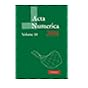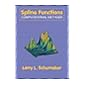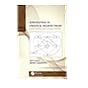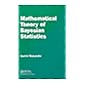Normal view MARC view ISBD view

Mathematical theory of bayesian statistics

Material type:BookPublisher: New York CRC Press 2018Description: ix, 319p. With index.ISBN: 9781482238068.DDC classification: 519.5​42 Summary: Mathematical Theory of Bayesian Statistics introduces the mathematical foundation of Bayesian inference which is well-known to be more accurate in many real-world problems than the maximum likelihood method. Recent research has uncovered several mathematical laws in Bayesian statistics, by which both the generalization loss and the marginal likelihood are estimated even if the posterior distribution cannot be approximated by any normal distribution.Explains Bayesian inference not subjectively but objectively. Provides a mathematical framework for conventional Bayesian theorems. Introduces and proves new theorems. Cross validation and information criteria of Bayesian statistics are studied from the mathematical point of view. Illustrates applications to several statistical problems, for example, model selection, hyper parameter optimization, and hypothesis tests.This book provides basic introductions for students, researchers, and users of Bayesian statistics, as well as applied mathematicians. https://www.crcpress.com/Mathematical-Theory-of-Bayesian-Statistics/Watanabe/p/book/9781482238068
Tags from this library: No tags from this library for this title.
average rating: 0.0 (0 votes)
Item type Current location Item location Collection Call number Status Date due Barcode
Books Vikram Sarabhai Library
General Stacks
Slot 1677 (2 Floor, East Wing) Non-fiction 519.5​42 W2M2 (Browse shelf) Available 198809
Browsing Vikram Sarabhai Library Shelves , Shelving location: General Stacks , Collection code: Non-fiction Close shelf browserNo cover image available No cover image available No cover image available 518.05 A2 Acta numerica Volume 10 2001 518.5 S2S7 Spline functions: computational methods 519.5​42 B2I6 Introduction to statistical decision theory: utility theory and causal analysis 519.5​42 W2M2 Mathematical theory of bayesian statistics 519 B2I6 An introduction to stochastic processes: with special reference to methods and applications 519 B5T4 Theory of game and statistical decisions 519 B7S8 Statistics and probability and their applications

Mathematical Theory of Bayesian Statistics introduces the mathematical foundation of Bayesian inference which is well-known to be more accurate in many real-world problems than the maximum likelihood method. Recent research has uncovered several mathematical laws in Bayesian statistics, by which both the generalization loss and the marginal likelihood are estimated even if the posterior distribution cannot be approximated by any normal distribution.Explains Bayesian inference not subjectively but objectively. Provides a mathematical framework for conventional Bayesian theorems. Introduces and proves new theorems.
Cross validation and information criteria of Bayesian statistics are studied from the mathematical point of view. Illustrates applications to several statistical problems, for example, model selection, hyper parameter optimization, and hypothesis tests.This book provides basic introductions for students, researchers, and users of Bayesian statistics, as well as applied mathematicians.

https://www.crcpress.com/Mathematical-Theory-of-Bayesian-Statistics/Watanabe/p/book/9781482238068

There are no comments for this item.